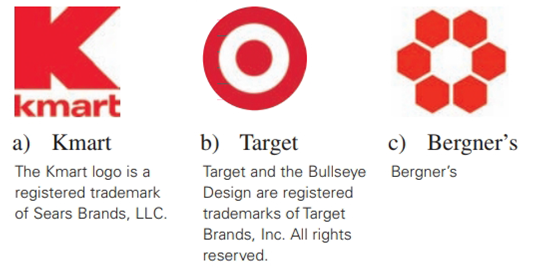Chapter 2.6, Problem 28E### Elementary Geometry for College St...

6th Edition
Daniel C. Alexander + 1 other
ISBN: 9781285195698

#### Solutions

Chapter
Section### Elementary Geometry for College St...

6th Edition
Daniel C. Alexander + 1 other
ISBN: 9781285195698
Textbook Problem
1 views

# Describe the type(s) of symmetry displayed by each of theseDepartment store logos.To determine

a)

The type(s) of symmetry displayed by given the logo.

Explanation

Given:

The given figure is,

Figure (1)

Definition Used:

(1) If for every point A on the figure there is a second point A on the figure for which line l is the perpendicular bisector of AA¯, then the figure has symmetry with respect to line l.

(2) If for every point A on the figure there is a second point A on the figure for which point P is the midpoint of AA¯, then the figure has symmetry with respect to point P

To determine

b)

The type(s) of symmetry displayed by given the logo.

To determine

c)

The type(s) of symmetry displayed by given the logo.

### Still sussing out bartleby?

Check out a sample textbook solution.

See a sample solution

#### The Solution to Your Study Problems

Bartleby provides explanations to thousands of textbook problems written by our experts, many with advanced degrees!

Get Started

#### 18. Compute .

Mathematical Applications for the Management, Life, and Social Sciences

#### Find the limit. limxln(sinx)

Single Variable Calculus: Early Transcendentals

#### Prove the fourth law of exponents [see (15)].

Single Variable Calculus: Early Transcendentals, Volume I

#### Evaluate the expression sin Exercises 116. (42)2

Finite Mathematics and Applied Calculus (MindTap Course List)

#### 2. Define the terms population, sample, parameter and statistic.

Statistics for The Behavioral Sciences (MindTap Course List)

#### Define a construct and explain the role that constructs play in theories.

Research Methods for the Behavioral Sciences (MindTap Course List)